# DH和几何法计算坐标一致性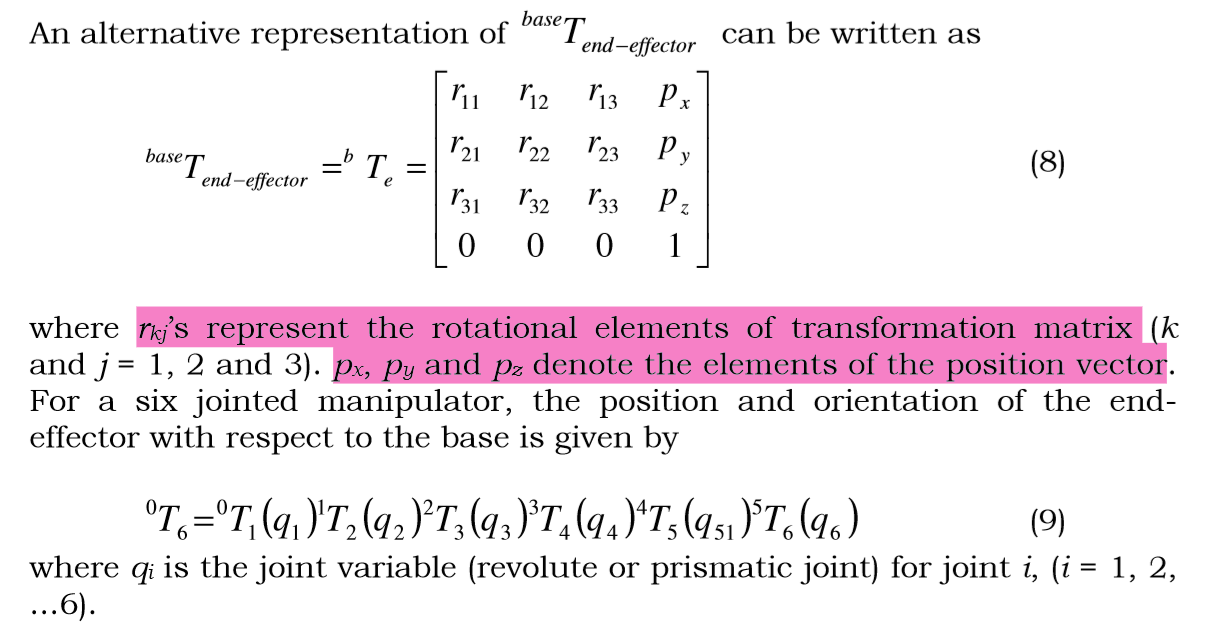# 思考

1. DH frame应怎么构建，尤其是X轴方向
2. DH参数如何取值
3. End-effector相对坐标如何表示

# Case Studies

## RR serial manipulator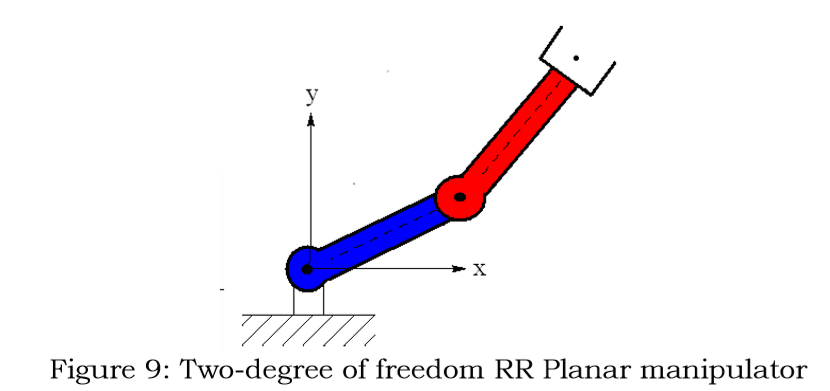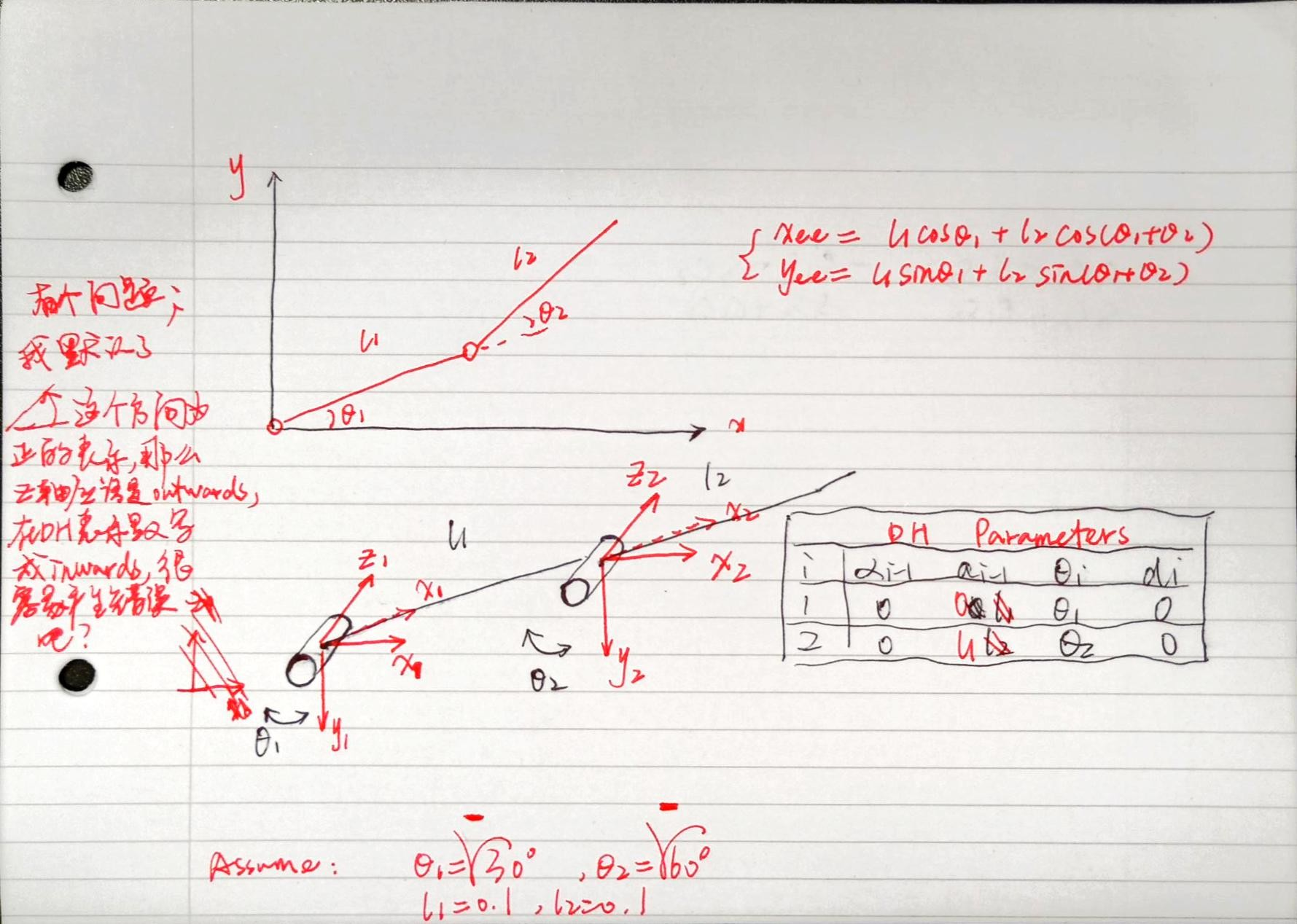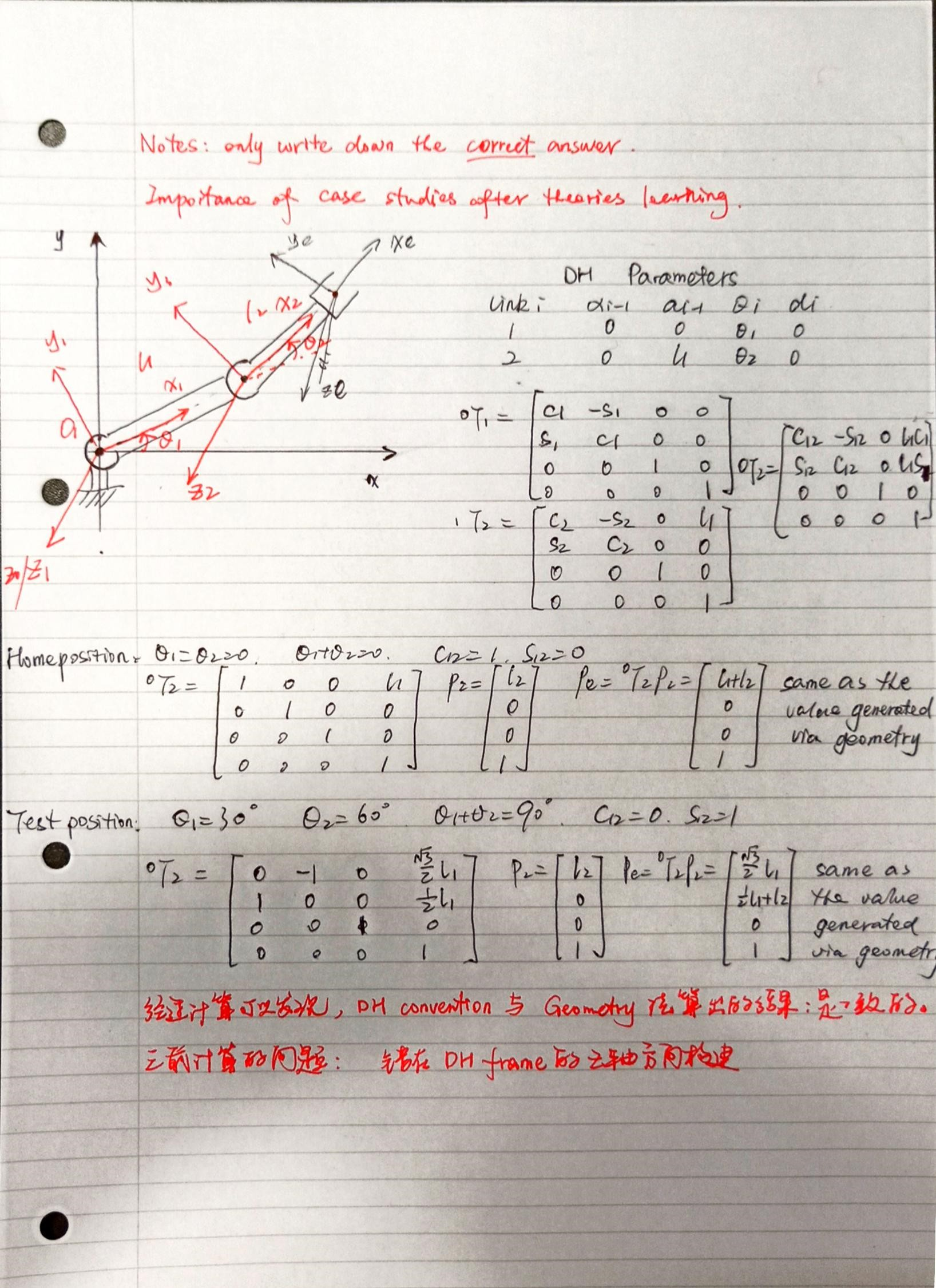1. $Z_0$最好是和$Z_1$一致，避免分析混乱

The base frame {0} is arbitrary. For simplicity chose $Z_0$ along $Z_1$ axis when the first joint variable is zero.

2. $X_0$沿着$Z_i$和$Z_{i+1}$之间的公共法线，而不是看$\theta$是不是0哦！

Assign $X_i$ axis pointing along the common normal ($a_i$) in the direction from $Z_i$ axis to $Z_{i+1}$ axis. In the case of $a_i=0$, $x_i$ is normal to the plane of $Z_i$ and $Z_{i+1}$ axes.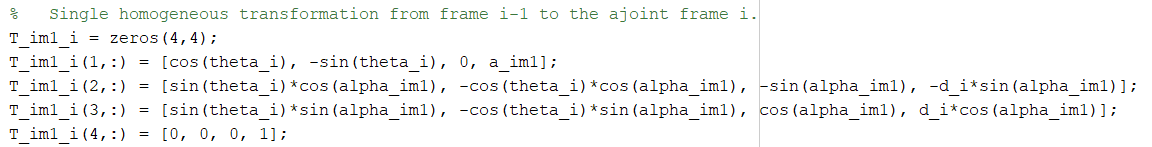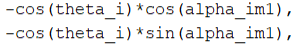## RRRR serial manipulator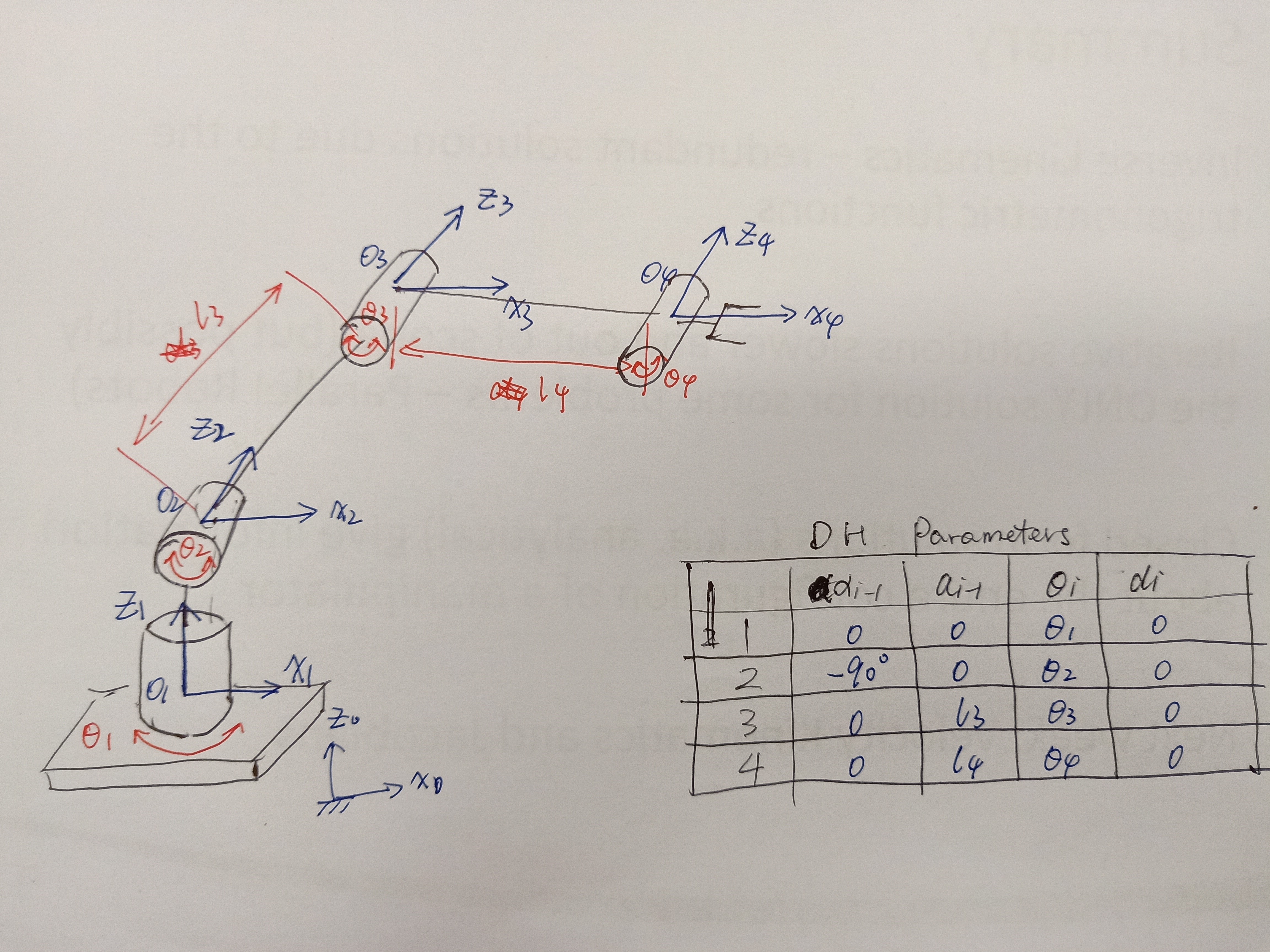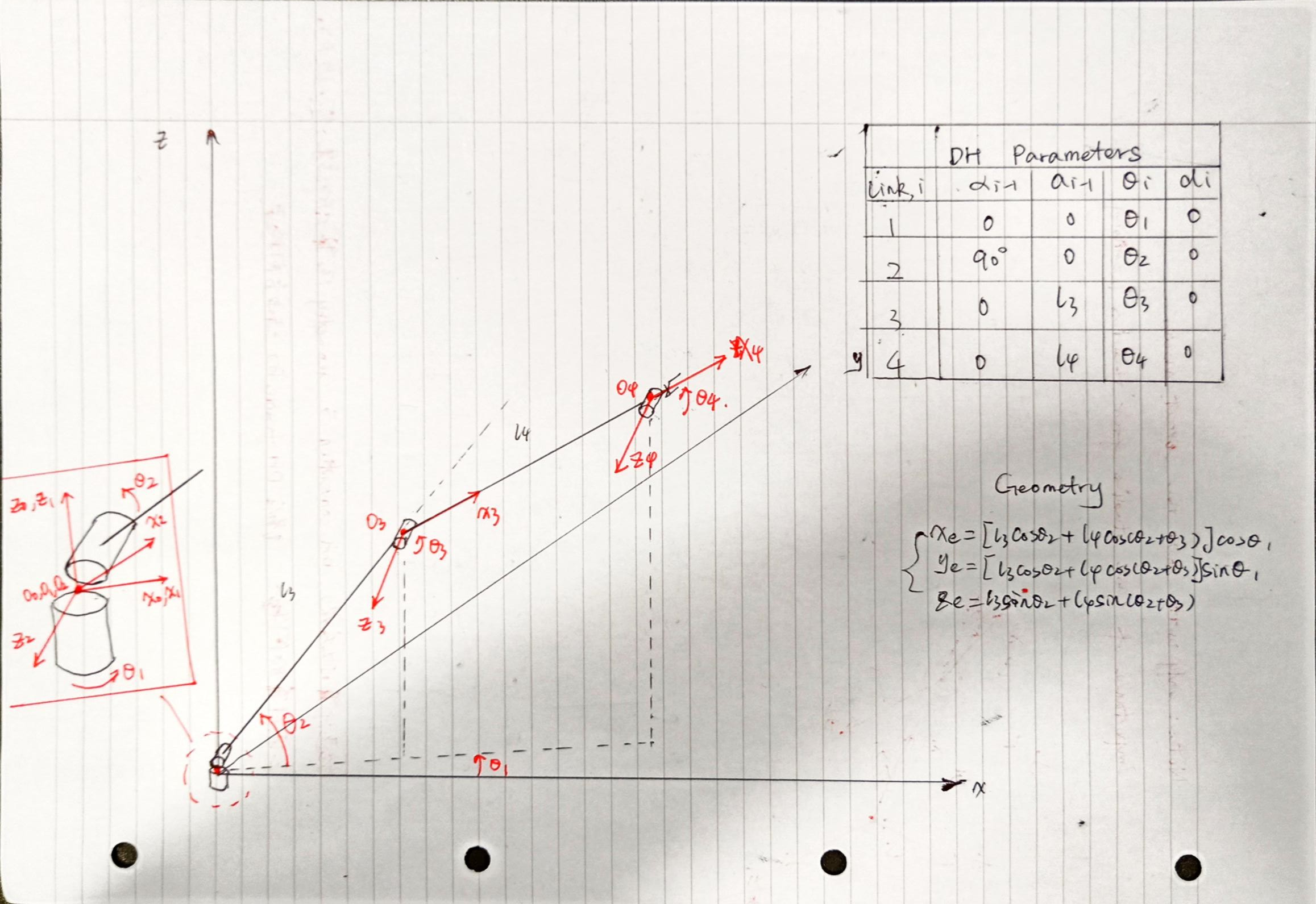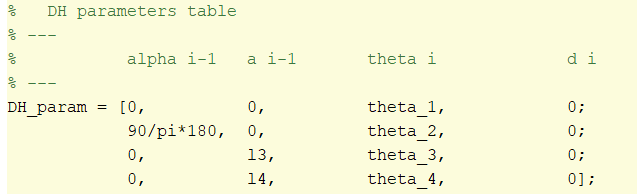90/pi*180，我写的啥代码啊。。。以后还不如90/180*pi这样好看懂。

## Case studies conclusion

1. 代码写错了（角度表示，方向和角度制转换）
2. DH convention的Z/X轴及角度和Geometry未统一

• 首先建模一定要正确，图形要画好，大方，规整，色彩分明

• 矩阵数据要利用tab排成矩阵型

# MATLAB 代码 (RRRR)

  1 2 3 4 5 6 7 8 9 10 11 12 13 14 15 16 17 18 19 20 21 22 23 24 25 26 27 28 29 30 31 32 33 34 35 36 37 38  % File: CalcFK.m % Name: Jinhang Zhu % Date: 25 Oct. 2019 % Email: lm19073@bristol.ac.uk % Descr: Calculate the homogeneous transformation matrix for modified DH convention. %% test 4R serial manipulator DH matrix theta_1 = 20*pi/180; theta_2 = 90*pi/180; theta_3 = 30*pi/180; theta_4 = -45*pi/180; l3 = 0.1; l4 = 0.1; % DH parameters table % --- % alpha i-1 a i-1 theta i d i % --- DH_param = [0, 0, theta_1, 0; 90*pi/180, 0, theta_2, 0; 0, l3, theta_3, 0; 0, l4, theta_4, 0]; % Matrix FK_mat = modified_DH_whole(DH_param); % Note that the end-effector position in frame 4 is at origin Pe = [0,0,0,1]'; Pe_DH = FK_mat *Pe; %% test geomerical location x_ee = (l3*cos(theta_2)+l4*cos(theta_2+theta_3))*cos(theta_1); y_ee = (l3*cos(theta_2)+l4*cos(theta_2+theta_3))*sin(theta_1); z_ee = l3*sin(theta_2)+l4*sin(theta_2+theta_3); xyz_ee = [x_ee,y_ee,z_ee]; %% compare disp('From DH convention'); Pe_DH(1:3,:) disp('From Geometry'); xyz_ee'first commit